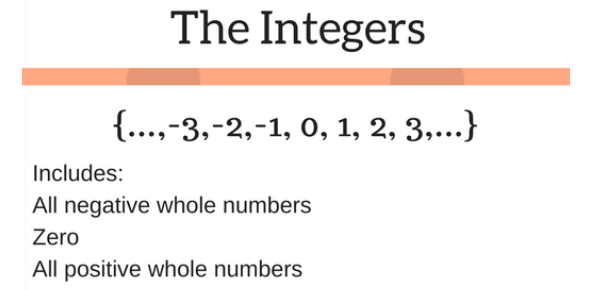# Integers Test: Math Trivia! Quiz

Approved & Edited by ProProfs Editorial Team
The editorial team at ProProfs Quizzes consists of a select group of subject experts, trivia writers, and quiz masters who have authored over 10,000 quizzes taken by more than 100 million users. This team includes our in-house seasoned quiz moderators and subject matter experts. Our editorial experts, spread across the world, are rigorously trained using our comprehensive guidelines to ensure that you receive the highest quality quizzes.
| By Combsk
C
Combsk
Community Contributor
Quizzes Created: 1 | Total Attempts: 694
Questions: 10 | Attempts: 699Settings.

• 1.

### 6 + -8 =

• A.

4

• B.

-2

• C.

2

• D.

0

B. -2
Explanation
When we add a positive number to a negative number, we subtract the absolute value of the smaller number from the absolute value of the larger number, and then assign the sign of the larger number to the result. In this case, adding 6 and -8 means subtracting the absolute value of -8 (which is 8) from the absolute value of 6 (which is 6), resulting in 2. Since -8 is the larger number and it is negative, the result is -2.

Rate this question:

• 2.

### Absolute value is distance from zero.

• A.

True

• B.

False

A. True
Explanation
The statement "Absolute value is distance from zero" is true. The absolute value of a number is the distance between that number and zero on the number line. It represents the magnitude or size of a number without considering its sign. For example, the absolute value of -5 is 5, as it is 5 units away from zero in the negative direction. Similarly, the absolute value of 5 is also 5, as it is 5 units away from zero in the positive direction. Therefore, the statement is correct.

Rate this question:

• 3.

### 8 - (-3) =

• A.

11

• B.

-11

• C.

5

• D.

-5

A. 11
Explanation
When subtracting a negative number, it is the same as adding the positive counterpart. In this case, we have 8 minus the negative 3. By adding the positive 3 to 8, we get 11. Therefore, the correct answer is 11.

Rate this question:

• 4.

### Integers does not include negative numbers.

• A.

True

• B.

False

B. False
Explanation
The statement "Integers does not include negative numbers" is incorrect. Integers include both positive and negative whole numbers, as well as zero. Therefore, the correct answer is False.

Rate this question:

• 5.

### -5 * -3 =

• A.

8

• B.

-4

• C.

15

• D.

-9

C. 15
Explanation
When multiplying two negative numbers, the result is always positive. In this case, -5 multiplied by -3 gives us a positive value. Therefore, the correct answer is 15.

Rate this question:

• 6.

### A negative integer multiplied by a negative integer produces a positive product.

• A.

True

• B.

False

A. True
Explanation
When two negative integers are multiplied together, the result is a positive product. This can be understood by considering the concept of multiplication as repeated addition. For example, if we have -2 multiplied by -3, it can be interpreted as adding -2 three times: -2 + (-2) + (-2) = -6. Similarly, if we have -3 multiplied by -4, it can be interpreted as adding -3 four times: -3 + (-3) + (-3) + (-3) = -12. In both cases, we can observe that the product is positive. Therefore, the statement is true.

Rate this question:

• 7.

### 9/-3 =

• A.

0

• B.

3

• C.

-6

• D.

-3

D. -3
Explanation
When dividing 9 by -3, the result is -3. This is because when a positive number is divided by a negative number, the quotient is negative. In this case, 9 divided by -3 equals -3.

Rate this question:

• 8.

### The rule for the subtraction of integers is to add the opposite.

• A.

True

• B.

False

A. True
Explanation
The rule for subtracting integers is to add the opposite. This means that when subtracting integers, you can change the subtraction sign to addition and change the sign of the number being subtracted to its opposite. By doing this, you can then add the two numbers together. Therefore, the statement "The rule for the subtraction of integers is to add the opposite" is true.

Rate this question:

• 9.

### -12 + -3 =

• A.

15

• B.

-15

• C.

9

• D.

-9

B. -15
Explanation
The correct answer is -15 because when you add a negative number to another negative number, the result is a negative number. In this case, -12 plus -3 equals -15.

Rate this question:

• 10.

### A positve integer divided by a negative integer gives a positive quotient.

• A.

True

• B.

False

B. False
Explanation
When a positive integer is divided by a negative integer, the quotient will be negative. This is because dividing a positive number by a negative number results in a negative value. Therefore, the statement that a positive integer divided by a negative integer gives a positive quotient is incorrect.

Rate this question:

Related TopicsBack to top